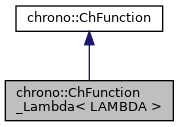chrono::ChFunction_Lambda< LAMBDA > Class Template Reference

## Description

### template<typename LAMBDA> class chrono::ChFunction_Lambda< LAMBDA >

Child of ChFunction designed to take a C++14 generic lambda as an argument. It provides analytical first and second derivatives via Dual Number forward-mode automatic differentiation, provided by the third party "yafel" finite element library. (https://github.com/tjolsen/YAFEL)

Due to the template on the generic lambda type, it is difficult to construct this class in the usual manner. For this reason, a function called "make_ChFunction_Lambda" has been provided to leverage modern C++ automatic return type deduction.

For similar reasons, a utility function called "make_shared_ChFunction_Lambda" is provided that returns a shared pointer to a heap-allocated ChFunction_Lambda.

Example usage:

auto F = make_ChFunction_Lambda( [](auto x) { return x*x; } );

auto Fp = make_shared_ChFunction_Lambda( [](auto x) { return x*x; } );

Template Parameters
 LAMBDA Type of generic lambda passed to constructor.

#include <ChFunction_Lambda.h>

Inheritance diagram for chrono::ChFunction_Lambda< LAMBDA >:[legend]
Collaboration diagram for chrono::ChFunction_Lambda< LAMBDA >:[legend]

## Public Member Functions

ChFunction_Lambda (LAMBDA &&_f)

virtual ChFunction_LambdaClone () const override
"Virtual" copy constructor.

virtual double Get_y (double x) const override
Return the y value of the function, at position x.

virtual double Get_y_dx (double x) const override
Return the dy/dx derivative of the function, at position x. More...

virtual double Get_y_dxdx (double x) const override
Return the ddy/dxdx double derivative of the function, at position x. More...

virtual FunctionType Get_Type () const override
Return the unique function type identifier.Public Member Functions inherited from chrono::ChFunction
ChFunction (const ChFunction &other)

virtual double Get_y_dxdxdx (double x) const
Return the dddy/dxdxdx triple derivative of the function, at position x. More...

virtual double Get_weight (double x) const
Return the weight of the function (useful for applications where you need to mix different weighted ChFunctions)

virtual void Estimate_x_range (double &xmin, double &xmax) const
Return an estimate of the range of the function argument. More...

virtual void Estimate_y_range (double xmin, double xmax, double &ymin, double &ymax, int derivate) const
Return an estimate of the range of the function value. More...

virtual double Get_y_dN (double x, int derivate) const
Return the function derivative of specified order at the given point. More...

virtual void Update (double x)
Update could be implemented by children classes, ex. to launch callbacks.

virtual double Compute_max (double xmin, double xmax, double sampling_step, int derivate) const
Compute the maximum of y(x) in a range xmin-xmax, using a sampling method.

virtual double Compute_min (double xmin, double xmax, double sampling_step, int derivate) const
Compute the minimum of y(x) in a range xmin-xmax, using a sampling method.

virtual double Compute_mean (double xmin, double xmax, double sampling_step, int derivate) const
Compute the mean value of y(x) in a range xmin-xmax, using a sampling method.

virtual double Compute_sqrmean (double xmin, double xmax, double sampling_step, int derivate) const
Compute the square mean val. of y(x) in a range xmin-xmax, using sampling.

virtual double Compute_int (double xmin, double xmax, double sampling_step, int derivate) const
Compute the integral of y(x) in a range xmin-xmax, using a sampling method.

virtual double Get_Ca_pos () const
Computes the positive acceleration coefficient (inherited classes should customize this).

virtual double Get_Ca_neg () const
Compute the positive acceleration coefficient (inherited classes should customize this).

virtual double Get_Cv () const
Compute the speed coefficient (inherited classes must customize this).

virtual int HandleNumber () const
Return the number of handles of the function.

virtual bool HandleAccess (int handle_id, double mx, double my, bool set_mode)
Get the x and y position of handle, given identifier. More...

virtual void ArchiveOut (ChArchiveOut &marchive)
Method to allow serialization of transient data to archives.

virtual void ArchiveIn (ChArchiveIn &marchive)
Method to allow de-serialization of transient data from archives. More...

virtual int FilePostscriptPlot (ChFile_ps *m_file, int plotY, int plotDY, int plotDDY)
Plot function in graph space of the ChFile_ps postscript file where zoom factor, centering, color, thickness etc. More...

virtual int FileAsciiPairsSave (ChStreamOutAscii &m_file, double xmin=0, double xmax=1, int msamples=200)
Save function as X-Y pairs separated by space, with CR at each pair, into an ASCII file. More...

virtual void EvaluateIntervaldN (ChMatrixDynamic<> &data, double xmin, double xmax, double step, int der=0)
Sample function on entire interval, up to Nth derivative (der = 0: y, 1: y_dx, 2: y_dxdx, 3: y_dxdxdx). More...Public Types inherited from chrono::ChFunction
enum  FunctionType {
FUNCT_CUSTOM, FUNCT_CONST, FUNCT_CONSTACC, FUNCT_DERIVE,
FUNCT_FILLET3, FUNCT_INTEGRATE, FUNCT_MATLAB, FUNCT_MIRROR,
FUNCT_MOCAP, FUNCT_NOISE, FUNCT_OPERATION, FUNCT_OSCILLOSCOPE,
FUNCT_POLY, FUNCT_POLY345, FUNCT_RAMP, FUNCT_RECORDER,
FUNCT_REPEAT, FUNCT_SEQUENCE, FUNCT_SIGMA, FUNCT_SINE,
FUNCT_LAMBDA, FUNCT_CYCLOIDAL, FUNCT_BSPLINE, FUNCT_DOUBLES
}
Enumeration of function types.

## ◆ Get_y_dx()

template<typename LAMBDA >
 virtual double chrono::ChFunction_Lambda< LAMBDA >::Get_y_dx ( double x ) const
inlineoverridevirtual

Return the dy/dx derivative of the function, at position x.

Note that inherited classes may also avoid overriding this method, because this base method already provide a general-purpose numerical differentiation to get dy/dx only from the Get_y() function. (however, if the analytical derivative is known, it may better to implement a custom method).

Reimplemented from chrono::ChFunction.

## ◆ Get_y_dxdx()

template<typename LAMBDA >
 virtual double chrono::ChFunction_Lambda< LAMBDA >::Get_y_dxdx ( double x ) const
inlineoverridevirtual

Return the ddy/dxdx double derivative of the function, at position x.

Note that inherited classes may also avoid overriding this method, because this base method already provide a general-purpose numerical differentiation to get ddy/dxdx only from the Get_y() function. (however, if the analytical derivative is known, it may be better to implement a custom method).

Reimplemented from chrono::ChFunction.

The documentation for this class was generated from the following file:
• /builds/uwsbel/chrono/src/chrono/motion_functions/ChFunction_Lambda.h